# Box Plots¶

The following illustrates some options for the boxplot in statsmodels. These include violin_plot and bean_plot.

In :
from __future__ import print_function
%matplotlib inline

import numpy as np
import matplotlib.pyplot as plt
import statsmodels.api as sm


## Bean Plots¶

The following example is taken from the docstring of beanplot.

We use the American National Election Survey 1996 dataset, which has Party Identification of respondents as independent variable and (among other data) age as dependent variable.

In :
data = sm.datasets.anes96.load_pandas()
party_ID = np.arange(7)
labels = ["Strong Democrat", "Weak Democrat", "Independent-Democrat",
"Independent-Independent", "Independent-Republican",
"Weak Republican", "Strong Republican"]


Group age by party ID, and create a violin plot with it:

In :
plt.rcParams['figure.subplot.bottom'] = 0.23  # keep labels visible
plt.rcParams['figure.figsize'] = (10.0, 8.0)  # make plot larger in notebook
age = [data.exog['age'][data.endog == id] for id in party_ID]
fig = plt.figure()
plot_opts={'cutoff_val':5, 'cutoff_type':'abs',
'label_fontsize':'small',
'label_rotation':30}
sm.graphics.beanplot(age, ax=ax, labels=labels,
plot_opts=plot_opts)
ax.set_xlabel("Party identification of respondent.")
ax.set_ylabel("Age")
#plt.show()

Out:
Text(0, 0.5, 'Age')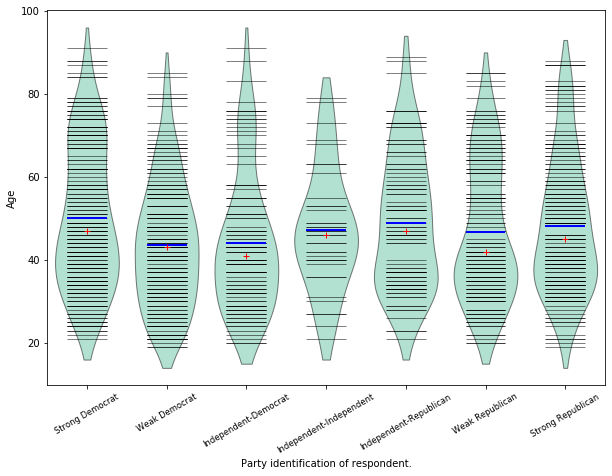In :
def beanplot(data, plot_opts={}, jitter=False):
"""helper function to try out different plot options
"""
fig = plt.figure()
plot_opts_ = {'cutoff_val':5, 'cutoff_type':'abs',
'label_fontsize':'small',
'label_rotation':30}
plot_opts_.update(plot_opts)
sm.graphics.beanplot(data, ax=ax, labels=labels,
jitter=jitter, plot_opts=plot_opts_)
ax.set_xlabel("Party identification of respondent.")
ax.set_ylabel("Age")

In :
fig = beanplot(age, jitter=True)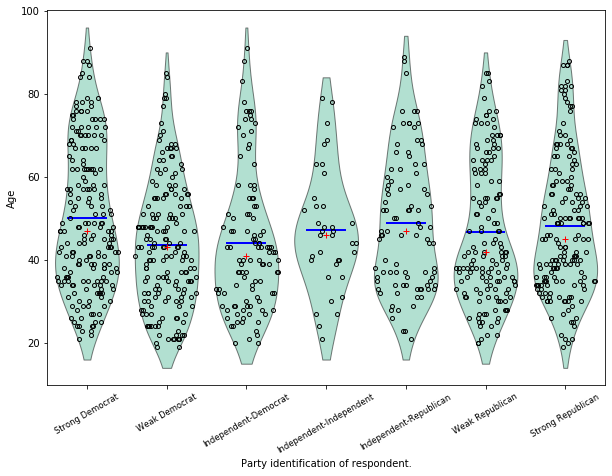In :
fig = beanplot(age, plot_opts={'violin_width': 0.5, 'violin_fc':'#66c2a5'})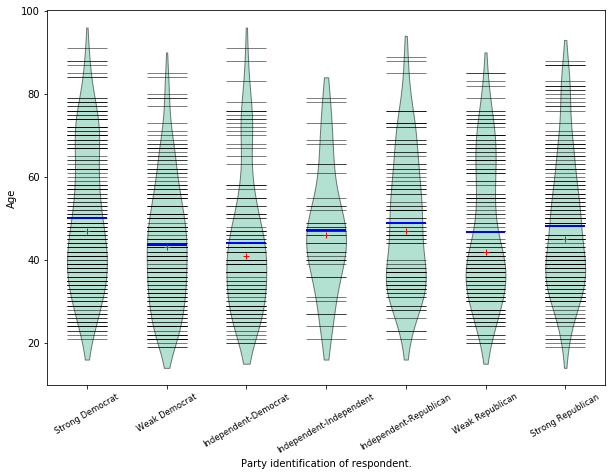In :
fig = beanplot(age, plot_opts={'violin_fc':'#66c2a5'})In :
fig = beanplot(age, plot_opts={'bean_size': 0.2, 'violin_width': 0.75, 'violin_fc':'#66c2a5'})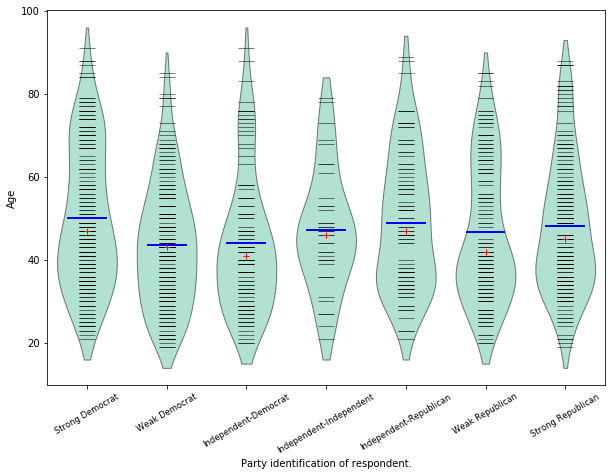In :
fig = beanplot(age, jitter=True, plot_opts={'violin_fc':'#66c2a5'})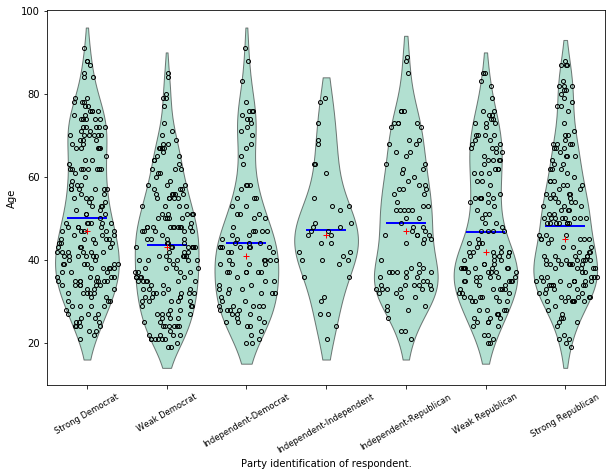In :
fig = beanplot(age, jitter=True, plot_opts={'violin_width': 0.5, 'violin_fc':'#66c2a5'})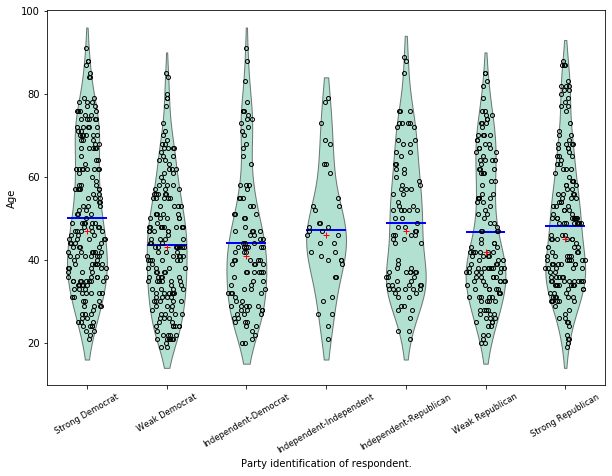In [ ]:



Based of example script example_enhanced_boxplots.py (by Ralf Gommers)

In :
import numpy as np
import matplotlib.pyplot as plt

import statsmodels.api as sm

# Necessary to make horizontal axis labels fit
plt.rcParams['figure.subplot.bottom'] = 0.23

party_ID = np.arange(7)
labels = ["Strong Democrat", "Weak Democrat", "Independent-Democrat",
"Independent-Independent", "Independent-Republican",
"Weak Republican", "Strong Republican"]

# Group age by party ID.
age = [data.exog['age'][data.endog == id] for id in party_ID]

In :
# Create a violin plot.
fig = plt.figure()

sm.graphics.violinplot(age, ax=ax, labels=labels,
plot_opts={'cutoff_val':5, 'cutoff_type':'abs',
'label_fontsize':'small',
'label_rotation':30})

ax.set_xlabel("Party identification of respondent.")
ax.set_ylabel("Age")
ax.set_title("US national election '96 - Age & Party Identification")

Out:
Text(0.5, 1.0, "US national election '96 - Age & Party Identification")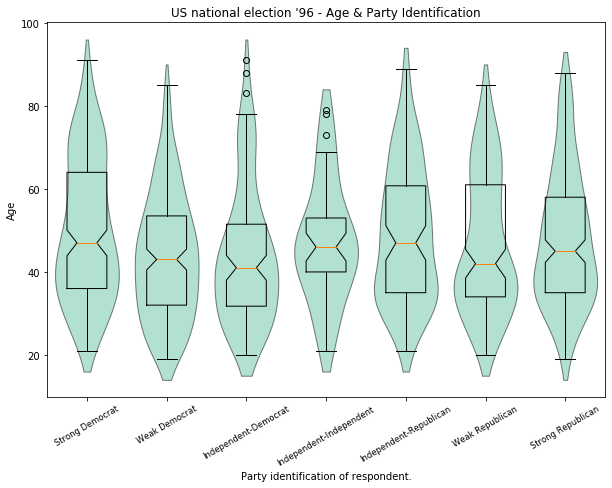In :
# Create a bean plot.
fig2 = plt.figure()

Text(0.5, 1.0, "US national election '96 - Age & Party Identification")